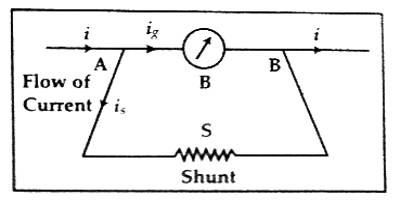Physics

# Use of Shunt in GalvanometerUse of Shunt in Galvanometer

The shunt is an electrical conductor joining two points of a circuit, through which more or less of a current may be diverted. It is a device which allows electric current to pass around another point in the circuit by creating a low resistance path. In order to convert a Galvanometer into an Ammeter, a very low resistance known as “shunt” resistance is connected in parallel to Galvanometer.

Suppose a resistance S of small value is connected between the two ends A and B parallel to the galvanometer of resistance G [Figure]. This S is the shunt. Let the principal current in the circuit be ‘i’. While reaching point ‘A’ this current will be divided into two parts. A small portion of the principal current will flow through the galvanometer and large current will flow through the shunt. As a result, the galvanometer will not be damaged due to heat produced for a large flow of current.Two currents will meet at ‘B’ and will form principal current again. Let this current through the galvanometer and the shunt be respectively ig and is. Now it the potential difference between the points A and B is (VA – VB), then according to Ohm’s law we get,

ig = (VA – VB) / G … … … (1)

is = (VA – VB) / S … …. …. (2)

Dividing equation (2) by equation (1), we get,

is / ig = G/S; or, is = ig x (G/S)

But, is + ig = i.

Inserting the value of is in this equation we get,

ig (G + S)/S = i

So, ig = (S x i) / (G + S) = principal current x (shunt resistance/total resistance) … … … (3)

or, i = ig x (G + S)/S; (G + S)/S, is called the power-multiplier of the shunt. .

Again, putting the value of ig in equation (3), we get,

is = (S x i) / (G + S) x G/S; or, S = (ig x G) / (i – ig)

So, is = (G x i) / (G x S) and, S = (ig x G) / (i – ig)

If 1/n th part of the principal current is to be sent through the galvanometer, then,

ig/i = 1/n = S / (G + S)

So, S = G / (n – 1), and, is = (n – 1)/n x i

Hence, by increasing n:

(1) Flow of current through galvanometer can be reduced and galvanometer can be protected from the heat generated due to flow of large current.

(2) It can be used to measure large current.